9.4 MARS: 多变量自适应回归样条¶

MARS 应该算是我接触得比较早的统计学习方法，大二的时候曾经在医学院某实验室搬砖，是关于基因数据的项目，具体项目记不太清，毕竟最后这个项目夭折了．但记得很清楚的是，我尝试使用 MARS 来建模，那时我还没有开始看 ESL，是在 Max Kuhn · Kjell Johnson 的 Applied Predictive Modeling 这本书中初识了 MARS．这本书介绍了一系列统计学习方法，以及如何利用 R 语言来实现．然后我就依葫芦画瓢学习了其中的例子，应用到了基因数据中，但基因数据实在太大了，跑啊跑啊，但由于种种原因，最后不了了之，与 MARS 挥手告别．

MARS 采用形式为 $(x-t)_+, (t-x)_+$ 的分段线性基函数的展开．“+”表示正的部分，所以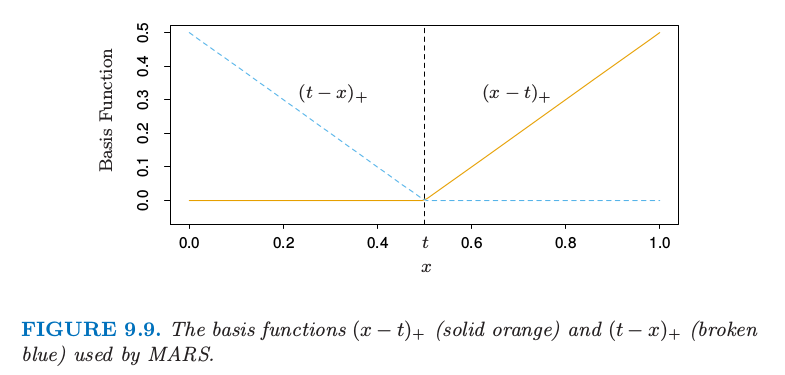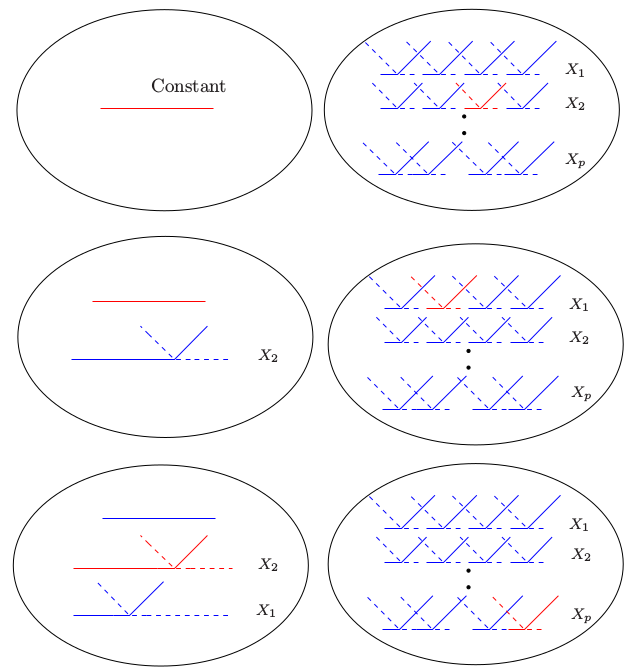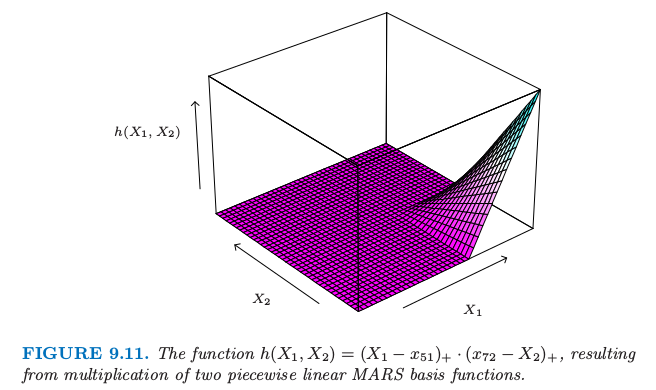weiya 注：$M(\lambda)=r+cK$?

@Xiaolin So@yvlian 在评论中讨论这个复杂度公式，由于理解不够透彻，有些回复可能有偏差，特此查阅MARS 原文试图在这里解释清楚.

Only the coefficients $(a_0, \ldots, a_M)$ (对应这里的 $(\beta_0,\ldots,\beta_M)$), the complexity cost function is

where $\B$ is the $M\times N$ data matrix of the $M$ (nonconstant) basis functions, $B_{ij} = B_i(\x_j)$. This is equal to the number of linearly independent basis functions.（这对应 7.10 节 中对 GCV 的近似 $\trace(\mathbf S)$, 其中 $\S$ 是线性算子）But with an increased cost complexity function $\tilde C(M)$ to reflect the additional (basis function) parameters that,

where $M$ is the number of nonconstant basis functions and $d$ represents a cost for each basis function optimization.

weiya 注

MARS 中向前建模的策略是层次性的，因为多重乘积是从已经在模型中的项来建立的．举个例子，仅仅当 4 重乘积中的一个 3 重乘积已经在模型中，才会加入该四重乘积．这里的哲学是，高阶交叉项仅仅当其中的低阶项存在才会存在．这个需求不是正确的，但是是合理的假设，并且避免了在可行选择数目呈指数增长的空间中的搜索．

MARS 过程中的一个有用选项是在交叉项的阶数上设置上界．举个例子，可以设置上界为 2，允许成对的分段线性函数的乘积，但是不允许 3 次或更高次的乘积．这个可以帮助最终模型的解释．含有上界得到加性模型．

垃圾邮件的例子（继续）¶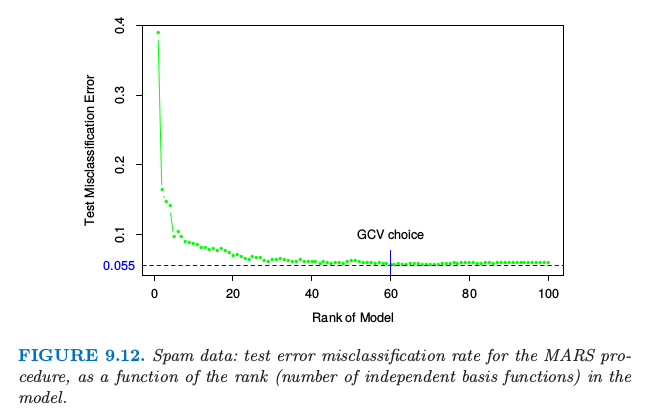例子（模拟数据）¶

情形3：¶

weiya 注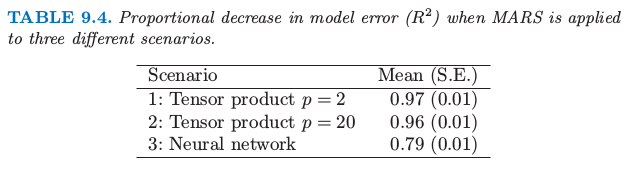weiya 注

其他的问题¶

MARS 用于分类¶

MARS 方法和算法可以拓展到处理分类问题．已经提出了几种策略．

Stone et al. (1997)1 发展了 MARS 的混合版本 PolyMARS，是为了处理分类问题特别设计的．它采用在 4.4 节描述的多重逻辑斯蒂回归框架．以类似 MARS 的向前逐步方式来建立模型，但是在每一步多多变量对数似然作二次近似来寻找下一个基函数对．一旦找到，则通过极大似然扩大模型，并且这个过程重复进行．

MARS 和 CART 的关系¶

• 用阶跃函数 $I(x-t>0)$ 和 $I(x-t\le 0)$ 来替换分段线性基函数．
• 当模型中的项与候选项相乘，替换成交叉项，因此不允许该项与其它候选项进行交叉．

混合输入¶

MARS 可以用一种自然的方式处理混合预测变量——定量和定性变量，这非常像 CART．对于一个定性变量，MARS 考虑将该定性变量的类别分成两块的所有可能的二叉分割．每个这样的分割产生成对分段常值基函数——关于两个类别集的指示函数．这个基对现在被看成和其他的一样，并且用它和其它已经在模型中的基函数来构造张量积．

1. Stone, M. (1977). An asymptotic equivalence of choice of model by crossvalidation and Akaike’s criterion, Journal of the Royal Statistical Society Series B. 39: 44–7.